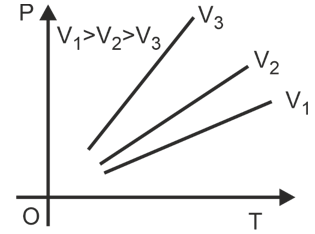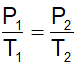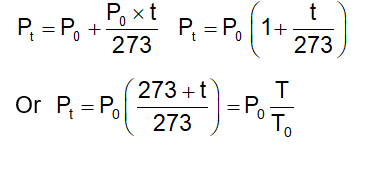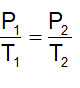# Formula For Gay Lussac Law

## Gay Lussac’s Law :

Gay Lussac's Law can be defined as the pressure of a given mass of any gas is directly proportional to its temperature in which volume remains constant . For More check Chemistry Formulas main page of Physics Wallah.Mathematically: P ∝ T (for constant n and v)

P/T = constant

If P1 and  P2 are volumes of a gas at temperature T1 and T2 respectively and the volume is kept constant, then:Graphically it is expressed as follows. Each line is called as Isochor. Note that slope is greater for lower volume. Volume remaining constant, the pressure of a given mass of a gas increases or decreases by 1/273 of its pressure at 0°C per degree change of temperature.or Pt/T = P0/T0   [P∝ T (if volume is kept constant)]

At constant volume, the pressure of a given amount of a gas is directly proportional to its absolute temperature.

### Solved Example of Gay Lussac’s Law

Q1. A certain amount of ethane is confined in a bulb of 1 liter capacity. The bulb is so weak that it will burst if pressure exceeds 10 atm. Initially gas exerts 8 atm pressure at 27°C. Find temperature at which the bulb will burst?

Ans. Considering limiting condition

Since volume remain constant =Thus 8/300= 10/T2

⇒T2 = 375 K